## 10-2 Discrete Fourier Transform (?¢æ•£?…ç??‰è???

In the previous section, we have introducde the discrete-time Fourier transform (DTFT) that transforms a discrete-time signal into a continuous function of its frequency components. Since DTFT is continuous in frequency, it is not suitable for digital processing by computers. In this section, we shall introduce discrete Fourier transform (DFT for short) that converts a discrete-time signals into its discrete-frequency components, which are suitable for further computer processing.

Suppose that the discrete-time signal can be expressed as x[n], n = 0~N-1. Then the formula for DFT is: $$X[k]=\frac{1}{N} \sum_{n=0}^{N-1} x[n] e^{-j2 \pi \frac{nk}{N}}, k=0, ..., N-1$$ The coefficient X[k] represents the amplitude and phase of the component of x[n], n = 0~N-1 at a specific frequency (to be detailed later). Therefore X[k], k = 0~N-1, are usually referred to as spectrum.

From X[k], we can also compute the original signal x[n], as follows: $$x[n]=\sum_{k=0}^{N-1}X[k] e^{j2\pi \frac{nk}{N}}, n=0, ..., N-1$$

Hint
DTFT and DFT are very similar; both are used to convert a discrete-time signal into its components at different frequencies. However, the former generates a continuous-frequency spectrum while the later produces a discrete-frequency spectrum.

Here we have several important facts about X[k]:

• If the length of the original signal x[n] is N, then the length of X[k] is also N.
• In general, X[k] is a complex number with a magnitude of |(X[k])| ¡]abs(X[k]) in MATLAB¡^ and a phase of ¡çX[k] (angle(X[k]) or atan(imag(X[k])/real(X[k]) in MATLAB).
• If the time-domain signal x[n] is real, then X[k] and X[N-k] are complex conjugate staisfying |(X[k])| = |(X[N-k])| and ¡çX[k] = -¡çX[N-k].
If x[n] is real, we can express it as follows:
x[n] = X
+ Xej2pn/N + X[N-1]ej2pn(N-1)/N
+ Xej2pn2/N + X[N-2]ej2pn(N-2)/N
+ Xej2pn3/N + X[N-3]ej2pn(N-3)/N
+ ...
For the terms involving k and N-k, we have
X[k]ej2pnk/N + X[N-k]ej2pn(N-k)/N
= X[k]ej2pnk/N + X[N-k]ej2pne-j2pnk/N
= X[k]ej2pnk/N + X[N-k]e-j2pnk/N
= 2 Re{X[k]ej2pnk/N}
If we use mk and pk to represent the magnitude and phase of X[k], respectively, then the above equation can be simplified as follows:
2 Re{mkexp(jpk)exp(j2pnk/N)}
= 2 Re{mkexp(j(2pnk/N + pk)}
= 2mkcos(2pnk/N + pk)
In general, N is even and we can express x[n] as
x[n] = X + 2Sk=1N/2-1mkcos(2pnk/N + pk) + mN/2cos(pn + pN/2)
The above equation states that we can decompose x[n] into a DC component X plus the summation of N/2 sinusoidal functions, where the amplitude and phase are determined by those of X[k], respectively. Therefore for a given real sequence x[n], we only need to have to observe X[k]¡Ak = 0 ~ N/2 for spectrum analysis. This "one-side spectrum" consists of 1+N/2 sinusoidal functions, with frequencies located at 0, fs/N, 2fs/N, 3fs/N, ..., N/2fs/N (=fs/2), with fs as the sample rate. These sinusoidal functions are referred as the fundamental sinusoids.

If we employ the above direct method for computing DFT, the time complexity if O(n2). In 1965, a smart method based on divide-and-conquer was proposed to compute DFT with a time complexity of O(n log n). The method is called fast Fourier transform (FFT for short). In other words, FFT is an efficient method for computing DFT.

First of all, let us use the MATLAB command fft to verify the conjugate property of the DFT coefficients:

Example 1: fftSym01.m% This example demonstrates the pair-wise conjugate of DFT (¦¹½d¨Ò®i¥Ü¹ê¼Æ°T¸¹¤§ DFT «Y¼Æªº¦@³m©Ê) N=64; % Length of vector x=randn(N, 1); z=fft(x); plot(z, 'o'); grid on %compass(z); % Connect conjugate pairs (±N¤W¤U¹ïºÙªº¦@³mÂI³s°_¨Ó) for i=2:N/2+1 twoPoint=z([i, N-i+2]); line(real(twoPoint), imag(twoPoint), 'color', 'r'); end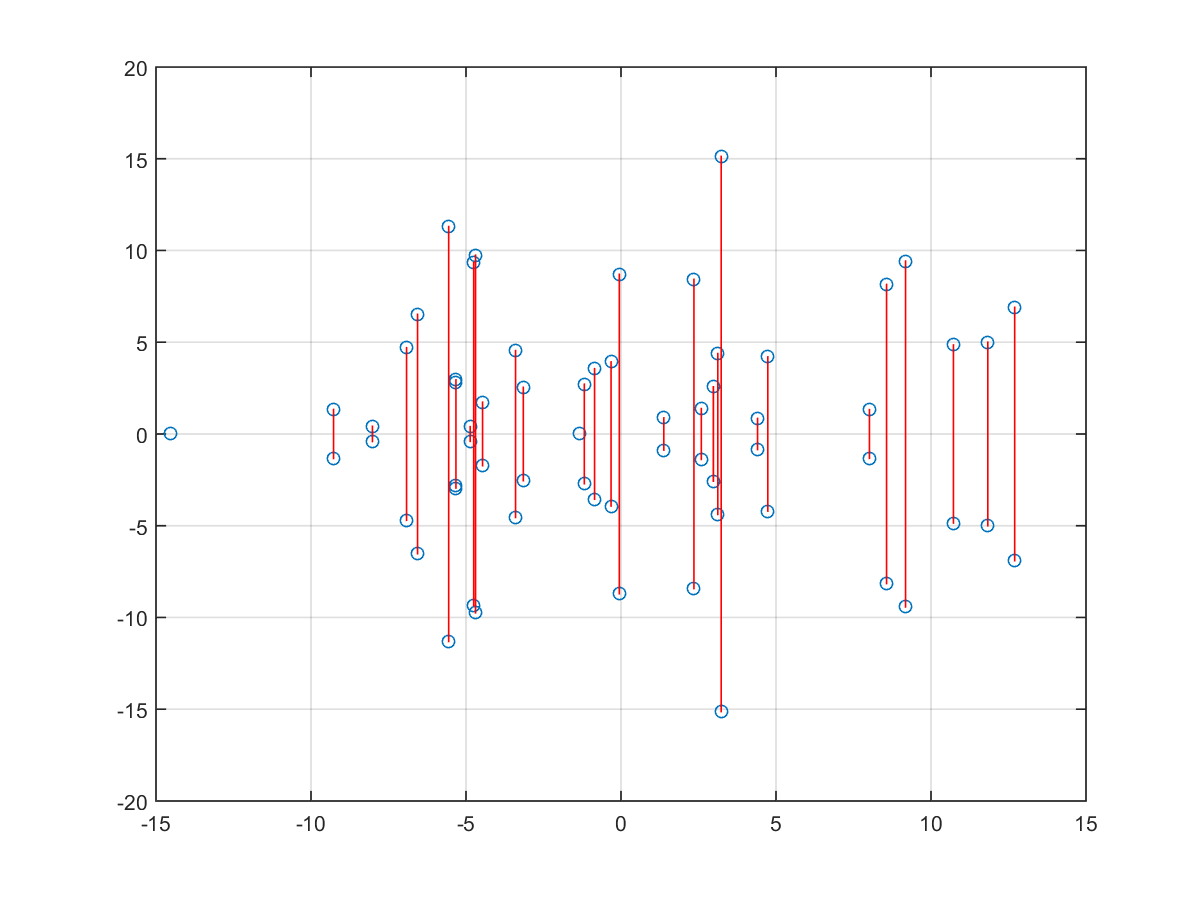From the above plot, it can be seen that the DFT coefficients appear as complex conjugate pairs, which have the x-axis as the symmetric axis.

Hint
For real sequence x[n], n = 0~N-1:
• If N is even, then X and X[N/2] are real and all the other terms are complex conjugate pairs.
• When N is odd, only X is real and all the other terms are complex conjugate pairs.

If x[n] happens to be one of the fundamental sinusoids, then the DFT coefficients should have only two non-zero terms, as shown in the following example:

Example 2: fft01.m% This example demonstrates the two-side DFT of a sinusoidal function % (¦¹½d¨Ò®i¥Ü¤@­ÓÂ²³æ¥¿©¶ªiªº³Å¥ß¸­Âà´«¡A¥HÂùÃäÀWÃÐ¨ÓÅã¥Ü) % Since the sinusoidal function has a frequency to be a multiple of fs/N, the two-side DFT have only two nonzero terms. % (¦¹¥¿©¶ªiªºÀW²v«ê¥©¬O freqStep ªº¾ã¼Æ­¿¡A©Ò¥HÂùÃäÀWÃÐÀ³¸Ó¥u¦³¨â­Ó«D¹sÂI) N = 256; % length of vector (ÂI¼Æ) fs = 8000; % sample rate (¨ú¼ËÀW²v) freqStep = fs/N; % freq resolution in spectrum (ÀW°ìªºÀW²vªº¸ÑªR«×) f = 10*freqStep; % freq of the sinusoid (¥¿©¶ªiªºÀW²v¡A«ê¬O freqStep ªº¾ã¼Æ­¿) time = (0:N-1)/fs; % time resolution in time-domain (®É°ìªº®É¶¡¨è«×) y = cos(2*pi*f*time); % signal to analyze Y = fft(y); % spectrum Y = fftshift(Y); % put zero freq at the center (±NÀW²v¶bªº¹sÂI¸m¤¤) % Plot time data subplot(3,1,1); plot(time, y, '.-'); title('Sinusoidal signals'); xlabel('Time (seconds)'); ylabel('Amplitude'); axis tight % Plot spectral magnitude freq = freqStep*(-N/2:N/2-1); % freq resolution in spectrum (ÀW°ìªºÀW²vªº¸ÑªR«×) subplot(3,1,2); plot(freq, abs(Y), '.-b'); grid on xlabel('Frequency)'); ylabel('Magnitude (Linear)'); % Plot phase subplot(3,1,3); plot(freq, angle(Y), '.-b'); grid on xlabel('Frequency)'); ylabel('Phase (Radian)');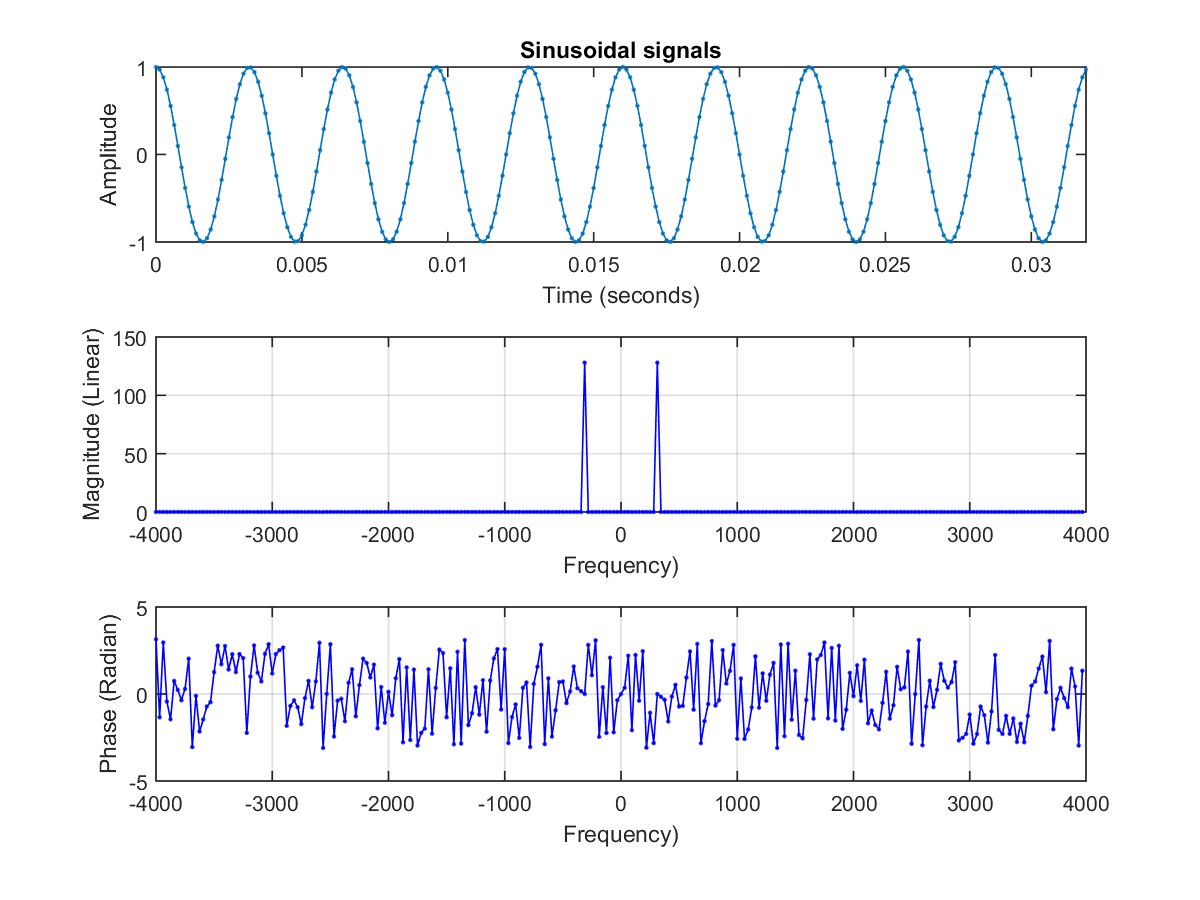We can observe that:
• In theory, the magnitude spectrum should have only two nonzero points. In practice, those zero points are not zero exactly, but very close to zero due to truncation or round-off errors.
• The phase spectrum appears to be random. This is simply because of the fact that most of the spectrum is zero, and hence the phase does not bear any significant meanings at all.
If x[n] is not one of the fundamental sinusoids, DFT will still decompose x[n] into the combination of fundamental sinusoids with spreaded magnitude spectrum:

Example 3: fft02.m% This example demonstrates the one-side DFT of a sinusoidal function (¦¹½d¨Ò®i¥Ü¤@­ÓÂ²³æ¥¿©¶ªiªº³Å¥ß¸­Âà´«¡A¥HÂùÃäÀWÃÐ¨ÓÅã¥Ü) % Since the sinusoidal function has a frequency not a multiple of fs/N, the two-side DFT smears. (¦¹¥¿©¶ªiªºÀW²v¤£¬O freqStep ªº¾ã¼Æ­¿¡A©Ò¥HÂùÃäÀWÃÐ·|¡u´²¶}¡v(Smearing)) N = 256; % length of vector (ÂI¼Æ) fs = 8000; % sample rate (¨ú¼ËÀW²v) freqStep = fs/N; % freq resolution in spectrum (ÀW°ìªºÀW²vªº¸ÑªR«×) f = 10.5*freqStep; % freq of the sinusoid (¥¿©¶ªiªºÀW²v¡A¤£¬O freqStep ªº¾ã¼Æ­¿) time = (0:N-1)/fs; % time resolution in time-domain (®É°ìªº®É¶¡¨è«×) signal = cos(2*pi*f*time); % signal to analyze [mag, phase, freq]=fftTwoSide(signal, fs, 1); % compute and plot the two-side DFT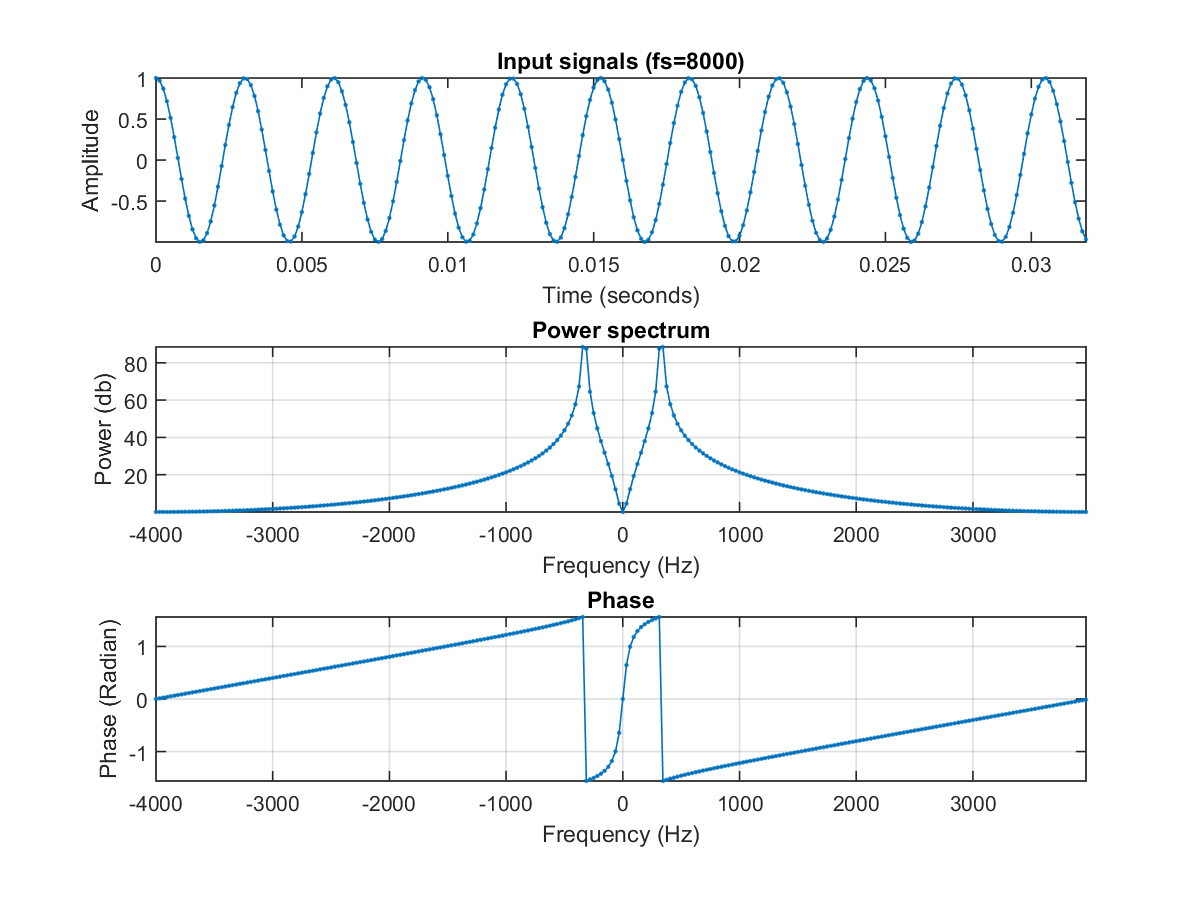In the above example, we have used the function fftTwoSide.m (in the SAP Toolbox) for the computation of two-side spectrum, and then plot the time-domain signal, magnitude spectrum and phase spectrum.

If x[n] is real, the magnitude spectrum is symmetric while the phase spectrum is anti-symmetric. Since we are dealing with audio signals which are all real, so we only need to have one-side spectrum for our analysis. In the following example, we shall use the function fftOneSide.m (in the SAP Toolbox) to plot the time-domain signal and one-side magnitude/phase spectrum:

Example 4: fft03.m% Same as fft02.m but use one-side DFT instead (¦P fft02.m¡A¦ý¥H³æÃäÀWÃÐ¨ÓÅã¥Ü) N = 256; % length of vector (ÂI¼Æ) fs = 8000; % sample rate (¨ú¼ËÀW²v) freqStep = fs/N; % freq resolution in spectrum (ÀW°ìªºÀW²vªº¸ÑªR«×) f = 10.5*freqStep; % freq of the sinusoid (¥¿©¶ªiªºÀW²v¡A¤£¬O freqStep ªº¾ã¼Æ­¿) time = (0:N-1)/fs; % time resolution in time-domain (®É°ìªº®É¶¡¨è«×) signal = cos(2*pi*f*time); % signal to analyze [mag, phase, freq]=fftOneSide(signal, fs, 1); % Compute and plot one-side DFT

Hint
Signals from the real world are all real, so we only need to use fftOneSide.m to obtain one-side spectrum for spectrum analysis.

In the following example, we shall demonstrate the one-side spectrum for a frame of an audio signal:

Example 5: fft04.m% Display DFT of a frame in a real-world audio signal (Åã¥Ü¤@­Ó»y­µ­µ®Øªº³æÃäÀWÃÐ) au=myAudioRead('welcome.wav'); frame=au.signal(2047:2047+237-1); [mag, phase, freq]=fftOneSide(frame, au.fs, 1);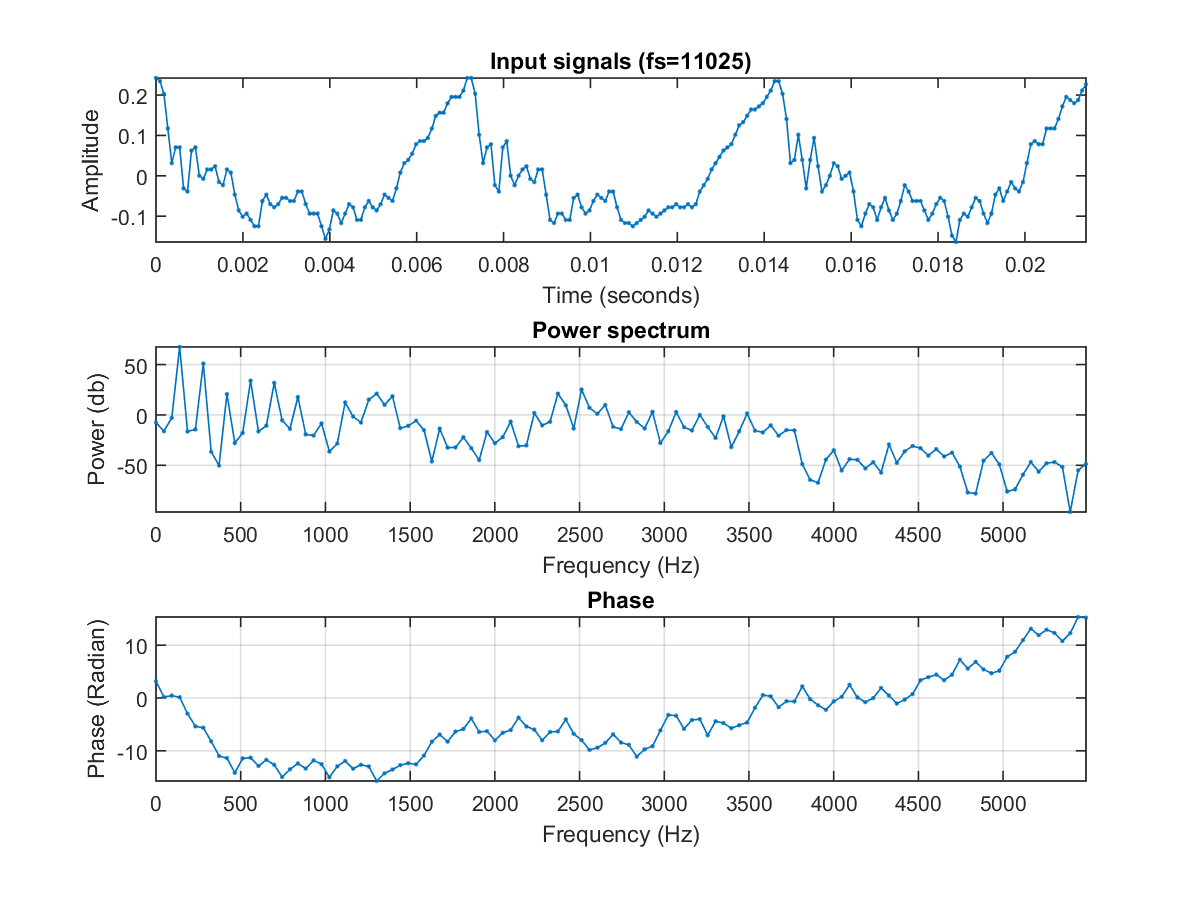In the above example, we can observe that there are harmonics in the magnitude spectrum. This is the result due to the fact that the time-domain signal is quasi-periodic. (To make the harmonics more obvious, we have carefully selected a time-domain signal containing three fundamental periods.)

When the value of k is getting bigger, we will have the high-frequency components which are more likely to be noises in the original time-domain signal. We can actually delete high-frequency components and use only low-frequency components to approximate and synthesize the original signal, to achieve the following goals:

• Data compression
• Low-pass filter
In the next example, we shall demonstrate how to synthesize a square wave using sinusolids:

Example 6: fftApproximate01.m% This example demos the effect of square wave approximation by DFT figure L = 15; N = 25; x = [ones(1,L), zeros(1,N-L)]; frameSize=length(x); runNum=3; for i=1:runNum, pointNum=ceil(frameSize/(2*runNum)*i); % Actually 2*pointNum-1 coefs are taken X = fft(x); magX = abs(X); remainIndex=[1:pointNum, frameSize-pointNum+2:frameSize]; X2=0*X; X2(remainIndex)=X(remainIndex); x2=ifft(X2); x2=real(x2); subplot(3,2,2*i-1); stem(x); hold on plot(x2, 'r'); hold off title(sprintf('x[n] and %d-points approximation', 2*pointNum-1)); axis([-inf,inf,-0.5,1.5]) subplot(3,2,2*i); shiftedMagX=fftshift(magX); plot(shiftedMagX, '.-'); axis tight title('DFT of x[n]') hold on temp=ifftshift(1:frameSize); ind=temp(remainIndex); plot(ind, shiftedMagX(ind), 'or'); grid on hold off end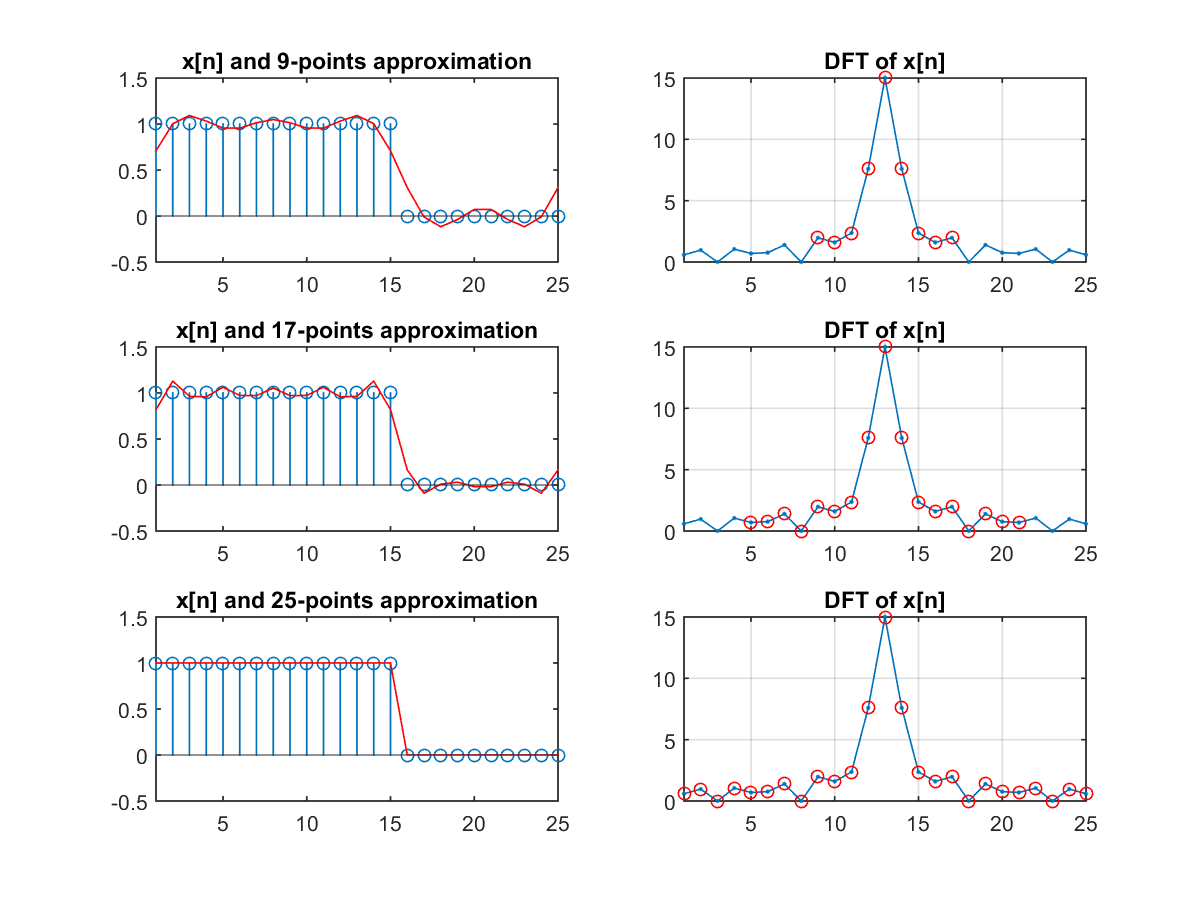It is obvious that when we use more DFT coefficients for the synthesis, the reconstructed waveform is closer to the original one.

The following example uses sinusoids to approximate the waveform of an utterance:

Example 7: fftApproximate02.m% Display the effect of FFT approximation au=myAudioRead('welcome.wav'); x=au.signal(2047:2047+237-1); figure frameSize=length(x); runNum=3; for i=1:runNum, pointNum=ceil(frameSize/(8*runNum)*i); % Actually 2*pointNum-1 coefs are taken X = fft(x); magX = abs(X); remainIndex=[1:pointNum, frameSize-pointNum+2:frameSize]; X2=0*X; X2(remainIndex)=X(remainIndex); x2=ifft(X2); x2=real(x2); subplot(3,2,2*i-1); plot(x, '.-'); hold on plot(x2, 'r'); hold off title(sprintf('x[n] and %d-points approximation', 2*pointNum-1)); set(gca, 'xlim', [-inf inf]); subplot(3,2,2*i); shiftedMagX=fftshift(magX); plot(shiftedMagX, '.-'); title('DFT of x[n]') hold on temp=ifftshift(1:frameSize); ind=temp(remainIndex); plot(ind, shiftedMagX(ind), 'or'); grid on hold off set(gca, 'xlim', [-inf inf]); end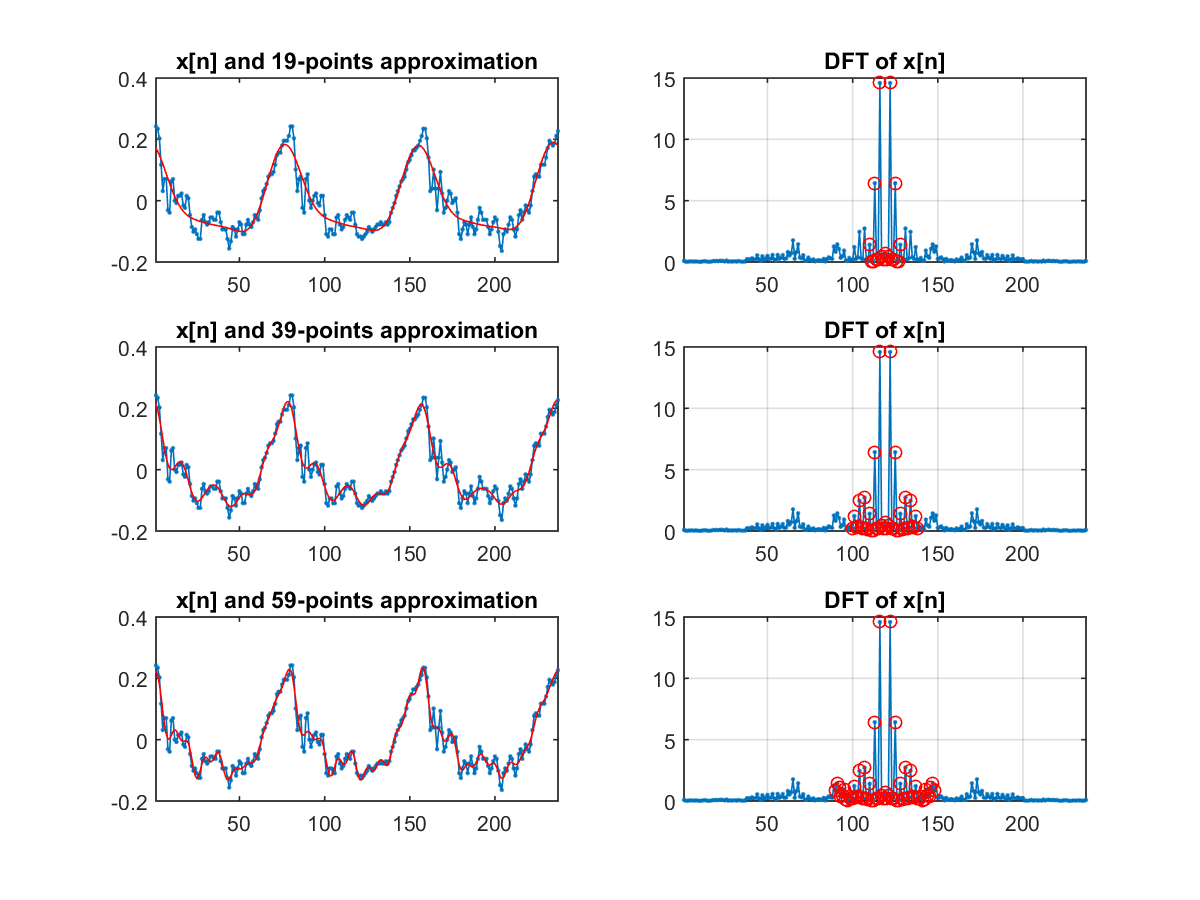From the above example, it is known that we only need a few DFT coefficents to synthesize the original signal with satisfactory quality. This fact demonstrate that the high-frequency components are not important in reconstructing the original signal.

For perfectly periodic signals, DFT will insert zeros inbetween, as follows:

Example 8: fftRepeat01.m% This example demos the effect of FFT for purely periodic signals au=myAudioRead('welcome.wav'); x=au.signal(2047:2126); % A full fundamental period runNum=5; for i=1:runNum repeatedX = x*ones(1,i); signal = repeatedX(:)+0.1; % signal=zeros(runNum*length(x), 1); % Zero-padding version % signal(1:length(repeatedX))=repeatedX(:); % Zero-padding version [mag, phase, freq, powerDb]=fftOneSide(signal, au.fs); mag=mag/length(signal); % Divided by vector length to normalize magnitude (due to the formula used by MATLAB) subplot(runNum,2,2*i-1); plot(signal, '.-'); grid on title('x[n]'); set(gca, 'xlim', [-inf inf]); subplot(runNum,2,2*i); plot(freq, mag, '.-'); grid on; % set(gca, 'yscale', 'log'); title('DFT of x[n]'); axis tight; end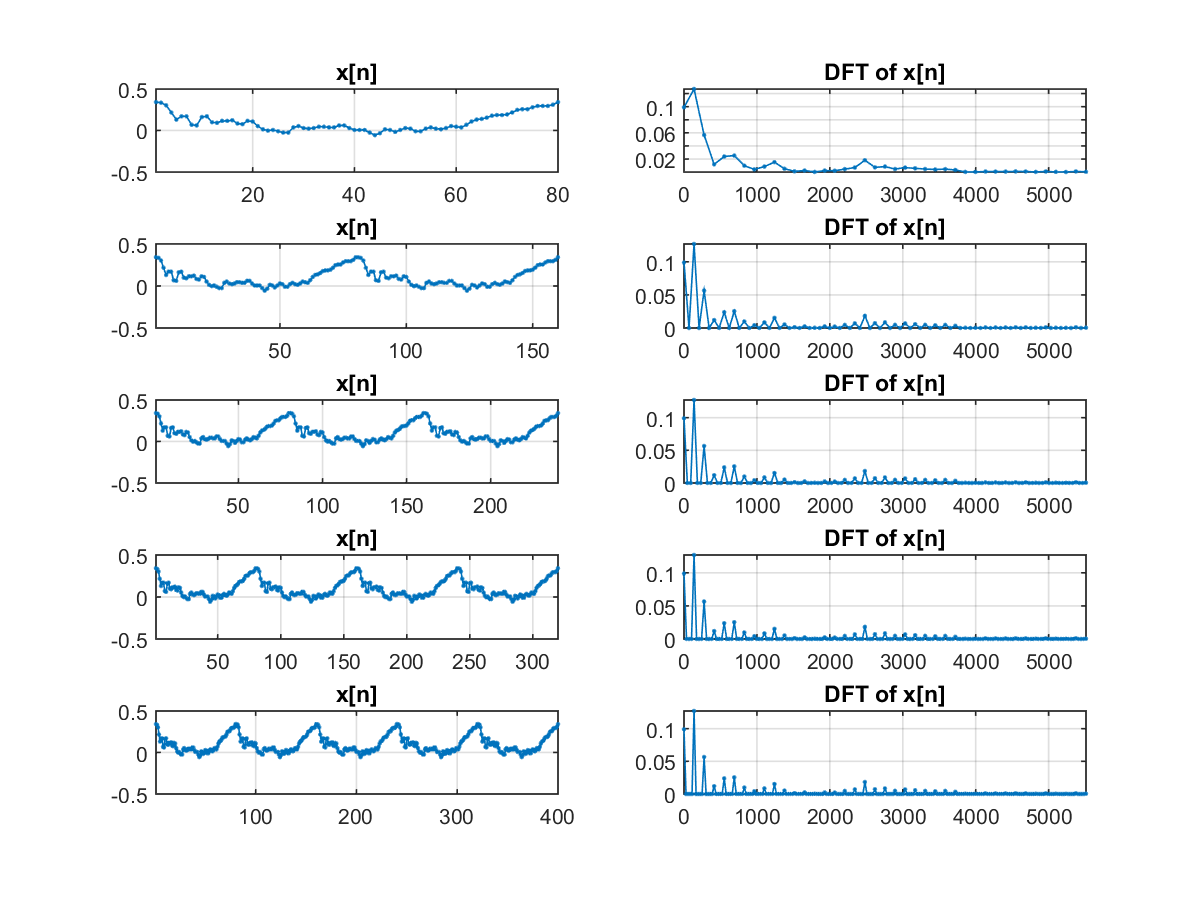Why is it so? Without rigorous analysis, can you explain this phonomenon by intuition?

If we pad the signal x[n] with zeros, the corresponding effect in frequency domain is interpolation, as shown in the next example:

Example 9: fftZeroPadding01.m% This example demos the effect of zero-padding of DFT for i=1:3 L = 5; N = 20*i; x = [ones(1,L), zeros(1,N-L)]; subplot(3,3,i*3-2); stem(x); title(sprintf('x[n] with N=%d',N)); set(gca, 'xlim', [-inf inf]); omega=((1:N)-ceil((N+1)/2))*(2*pi/N); X = fft(x); magX = fftshift(abs(X)); subplot(3,3,i*3-1); plot(omega, magX, '.-'); title('Magnitude of DFT of x[n]') set(gca, 'xlim', [-inf inf]); phase=fftshift(angle(X)); subplot(3,3,i*3); plot(omega, phase, '.-'); title('Phase of DFT of x[n]') set(gca, 'xlim', [-inf inf]); set(gca, 'ylim', [-pi pi]); end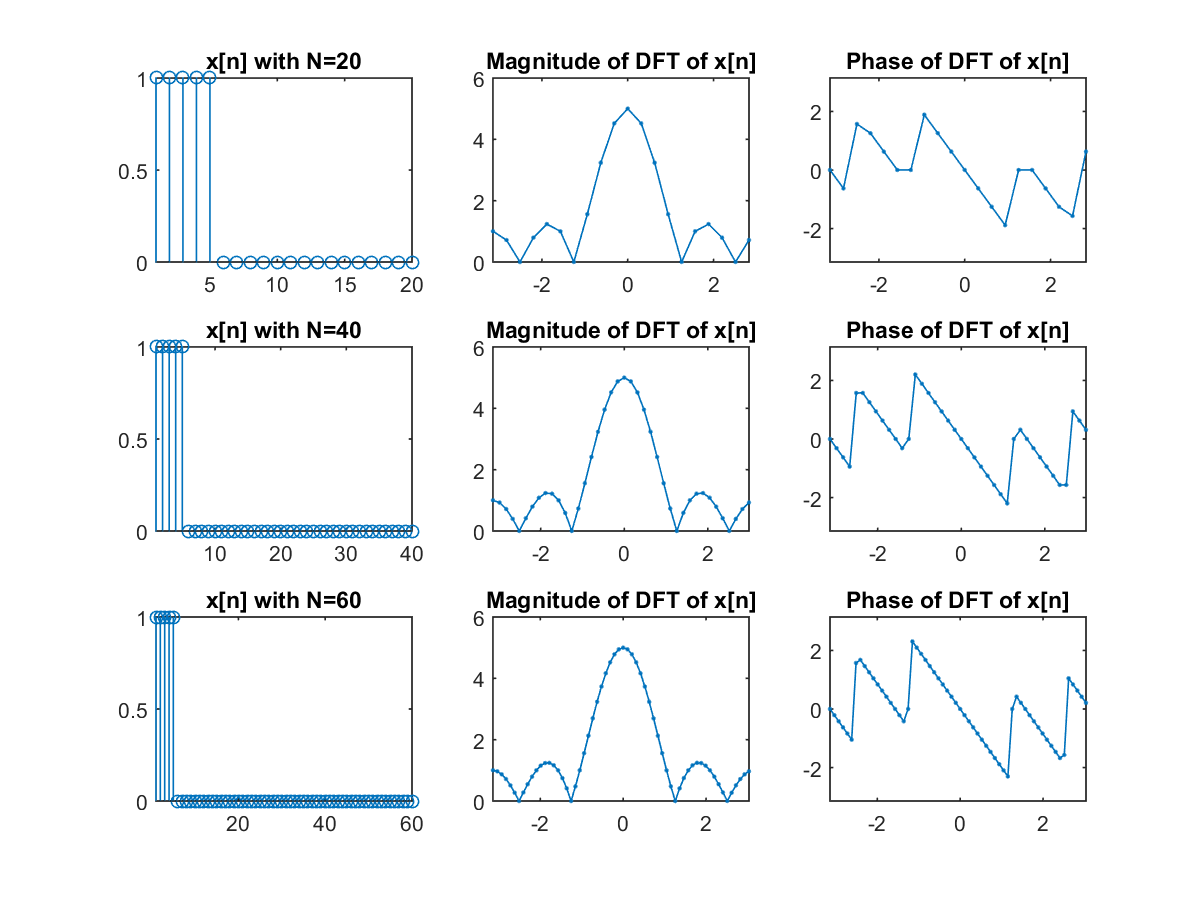In other words, zero padding does not add new information to x[n]. However, DFT need to have the same length and the net result is the interpolation to have a dense sampling in the frequency domain.

Hint
For common audio signal processing, if the frame size is not 2n, then we need to use zero padding until the frame size is 2n. This is also easier for DFT since a fast method based on 2-radix FFT can be invoked directly.

If we down sample the signal in the time domain, then the result in the spectrum is shown next:

Example 10: fftReSample01.m% This example demos the effect of FFT approximation au=myAudioRead('welcome.wav'); x=au.signal(2047:2126); x=au.signal(2047:2326); n=length(x); F = (0:n/2)*au.fs/n; runNum=5; for i=1:runNum, newX=x(1:2^(i-1):length(x)); newFs=au.fs/(2^(i-1)); X = fft(newX); magX = abs(X); frameSize=length(newX); subplot(runNum,2,2*i-1); plot(newX, '.-'); title('x[n]'); set(gca, 'xlim', [-inf inf]); subplot(runNum,2,2*i); freq = (0:frameSize/2)*newFs/frameSize; magX = magX(1:length(freq)); M=nan*F; M(1:length(magX))=magX; plot(F, M, '.-'); title('DFT of x[n]') axis tight; end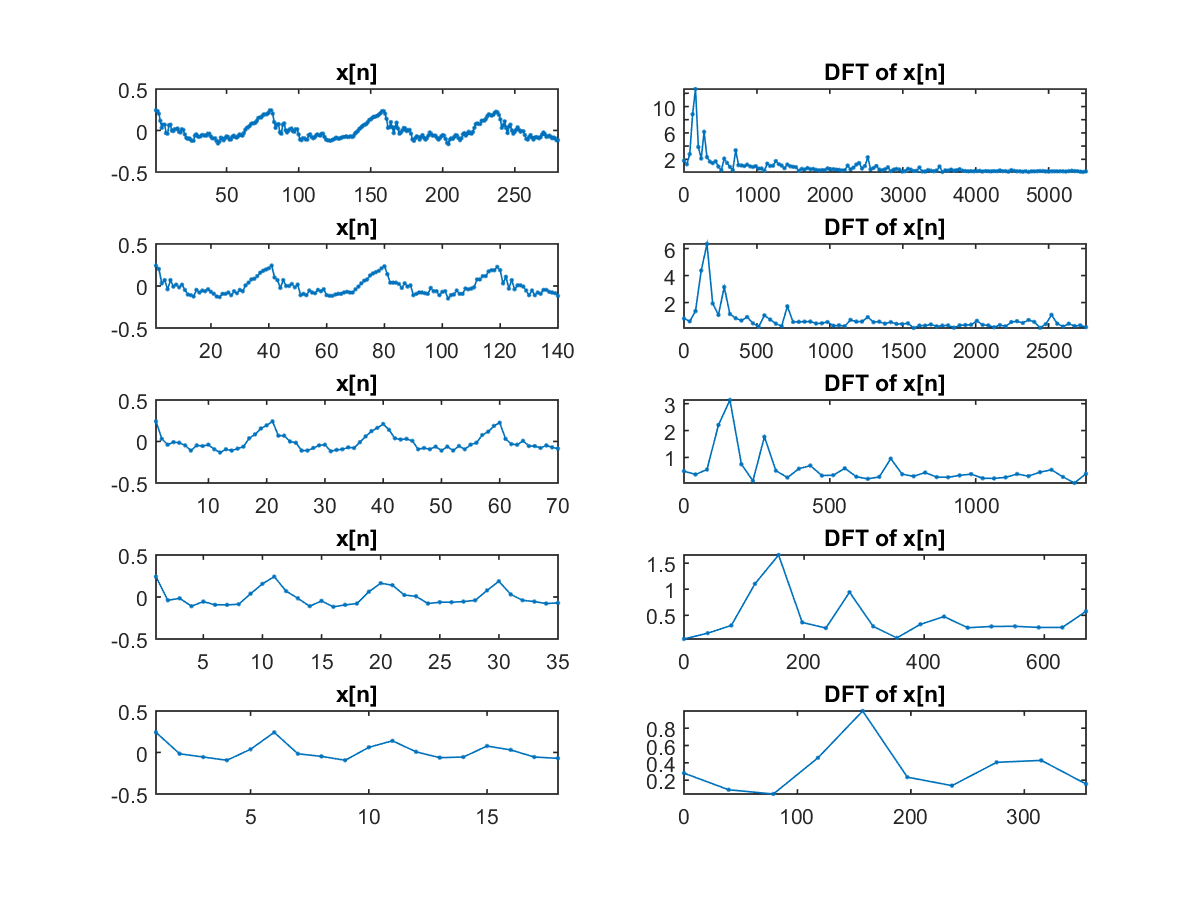The more times we do down sampling, the smoother the time-domain signal will be. Therefore the high-frequency components in the spectrum are missing gradually.

Moreover, we can express the time-domain signal as a linear combination of the fundamental sinusoids and then apply the least-square estimate to find the best coefficients of these sinusoids. It turns out that the coefficients identified by the least-square method are the same as those by fft, as shown next:

Example 11: fftViaLse01.m% FFT via least-squares estimate N=8; fs=1; time=(0:N-1)'/fs; x=rand(N,1)*2-1; A=ones(N,1); for i=1:N/2 A=[A, cos(2*pi*(i*fs/N)*time), sin(2*pi*(i*fs/N)*time)]; end th=A\x; plotNum=fix(N/2)+2; subplot(plotNum, 1, 1); N2=(N-1)*5+1; % Insert 4 points between every 2 points for better observation (¨âÂI¶¡´¡¤J¥|ÂI¡A¥H«KÆ[¹îªi§Î) timeFine=linspace(min(time), max(time), N2); x2=th(1)*ones(N,1); plot(timeFine, th(1)*ones(N2,1), '.-', time, x2, 'or'); % Plot the first term (µe¥X²Ä¤@¶µ) ylabel('Term 0 (DC)'); axis([0 N/fs -1 1]); grid on for i=1:N/2 % Plot terms 2 to 1+N/2 (µe¥X²Ä¤G¦Ü²Ä 1+N/2 ¶µ) freq=i*fs/N; y=th(2*i)*cos(2*pi*freq*time)+th(2*i+1)*sin(2*pi*freq*time); % a term (¨C¤@¶µ) x2=x2+y; fprintf('i=%d, sse=%f\n', i, norm(x-x2)/sqrt(N)); subplot(plotNum, 1, i+1); yFine=th(2*i)*cos(2*pi*(i*fs/N)*timeFine)+th(2*i+1)*sin(2*pi*(i*fs/N)*timeFine); % Finer verison for plotting plot(timeFine, yFine, '.-', time, y, 'or'); ylabel(sprintf('Term %d', i)); axis([0 N/fs -1 1]); grid on end % Plot the original signal (µe¥X­ì¨Ó°T¸¹) subplot(plotNum, 1, plotNum) plot(time, x, 'o-'); axis([0 N/fs -1 1]); grid on xlabel('Time'); ylabel('Orig signals'); % Transform LSE result back to fft format for comparison (±N th Âà¦^ fft ¨Ã¤ñ¸ûµ²ªG) F=fft(x); F2=[]; F2(1)=th(1)*N; for i=1:N/2 F2(i+1)=(th(2*i)-sqrt(-1)*th(2*i+1))*N/2; if (i==N/2) F2(i+1)=2*F2(i+1); end end % symmetric of DFT (DFT ªº¹ïºÙ©Ê) for i=N/2+2:N F2(i)=F2(N-i+2)'; end error1=sum(abs(F2-F.')); % F.' is simple transpose (F.' ¬O¤£¶i¦æ¦@³mÂà´«ªºÂà¸m) error2=sum(abs(F2-F')); % F' is conjugate transpose (F' ¬O¶i¦æ¦@³mÂà´«ªºÂà¸m) fprintf('Errors after transforming LSE to DFT coefficients (±N LSE Âà´«¦¨ DFT «Y¼Æªº»~®t)¡Gerror1=%f, error2=%f\n', error1, error2); fprintf('Due to the symmetry of DFT, one of the above error terms should be zero. (¥Ñ©ó DFT ªº¹ïºÙ©Ê¡A¤W­z»~®tÀ³¸Ó¦³¤@¶µ¬°¹s¡C)\n');i=1, sse=0.626965 i=2, sse=0.306336 i=3, sse=0.008109 i=4, sse=0.000000 Errors after transforming LSE to DFT coefficients (±N LSE Âà´«¦¨ DFT «Y¼Æªº»~®t)¡Gerror1=0.000000, error2=22.709014 Due to the symmetry of DFT, one of the above error terms should be zero. (¥Ñ©ó DFT ªº¹ïºÙ©Ê¡A¤W­z»~®tÀ³¸Ó¦³¤@¶µ¬°¹s¡C)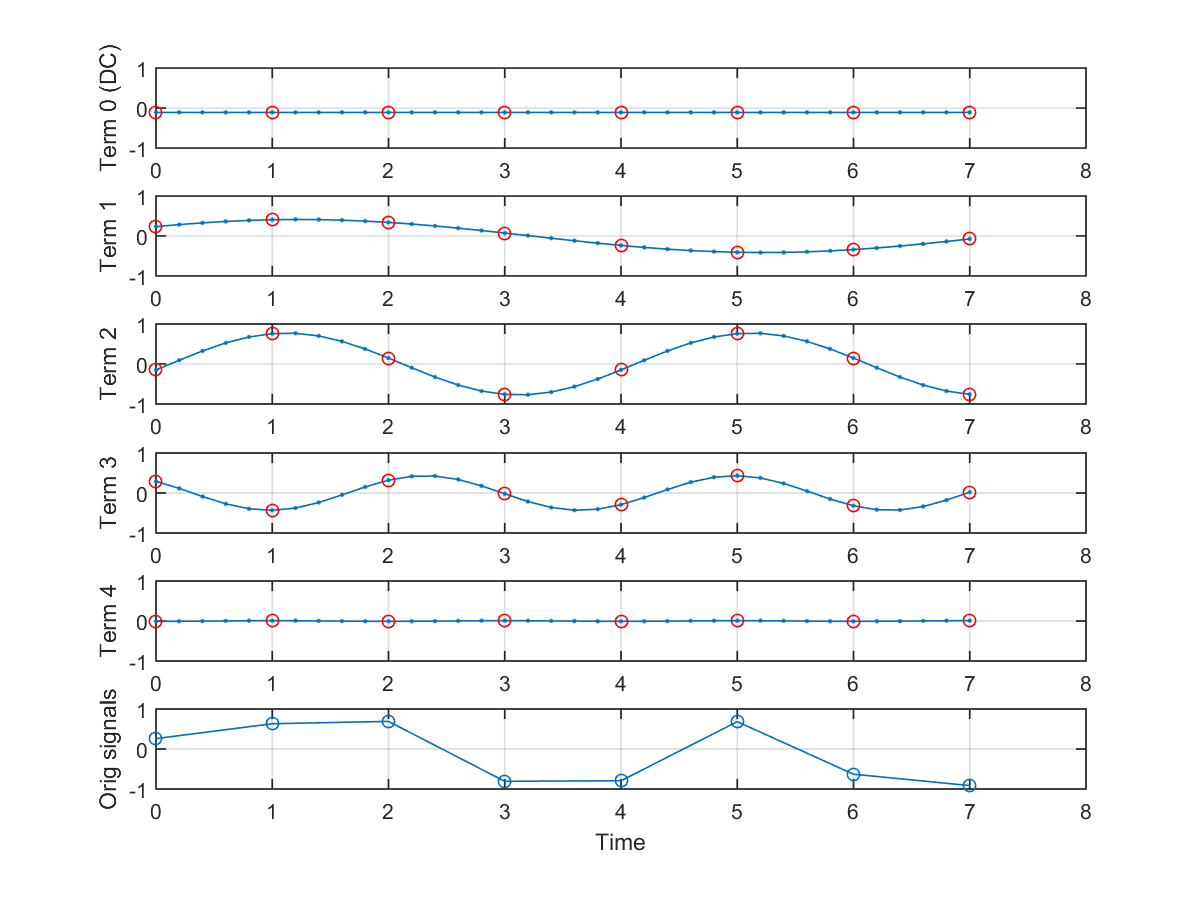Audio Signal Processing and Recognition (­µ°T³B²z»P¿ëÃÑ)